# Bernstein-Bézier form

(diff) ← Older revision | Latest revision (diff) | Newer revision → (diff)

Bernstein form, Bézier polynomial

The Bernstein polynomial of orderfor a function, defined on the closed interval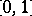, is given by the formula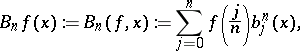with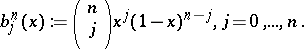The polynomial was introduced in 1912 (see, e.g., [a3]) by S.N. Bernstein (S.N. Bernshtein) and shown to converge, uniformly on the intervalas, toin caseis continuous, thus providing a wonderfully short, probability-theory based, constructive proof of the Weierstrass approximation theorem (cf. Weierstrass theorem).

The Bernstein polynomialis of degree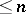and agrees within caseis a polynomial of degree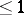. It depends linearly onand is positive onin caseis positive there, and so has served as the starting point of the theory concerned with the approximation of continuous functions by positive linear operators (see, e.g., [a1] and Approximation of functions, linear methods), with the Bernstein operator,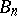, the prime example. See also Bernstein polynomials.

The-sequence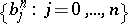is evidently linearly independent, hence a basis for the-dimensional linear spaceof all polynomials of degreewhich contains it. It is called the Bernstein–Bézier basis, or just the Bernstein basis, and the corresponding representation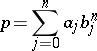is called the Bernstein–Bézier form, or just the Bernstein form, for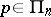. Thanks to the fundamental work of P. Bézier and P. de Casteljau, this form has become the standard way in computer-aided geometric design (see, e.g., [a2]) for representing a polynomial curve, that is, the image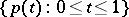of the intervalunder a vector-valued polynomial. The coefficientsin that form readily provide information about the value ofand its derivatives at both endpoints of the interval, hence facilitate the concatenation of polynomial curve pieces into a more or less smooth curve.

Somewhat confusingly, the term "Bernstein polynomial" is at times applied to the polynomial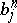, the term "Bézier polynomial" is often used to refer to the Bernstein–Bézier form of a polynomial, and, in the same vein, the term "Bézier curve" is often used for a curve that is representable by a polynomial, as well as for the Bernstein–Bézier form of such a representation.

How to Cite This Entry:
Bernstein-Bézier form. Encyclopedia of Mathematics. URL: http://encyclopediaofmath.org/index.php?title=Bernstein-B%C3%A9zier_form&oldid=12511
This article was adapted from an original article by C. de Boor (originator), which appeared in Encyclopedia of Mathematics - ISBN 1402006098. See original article